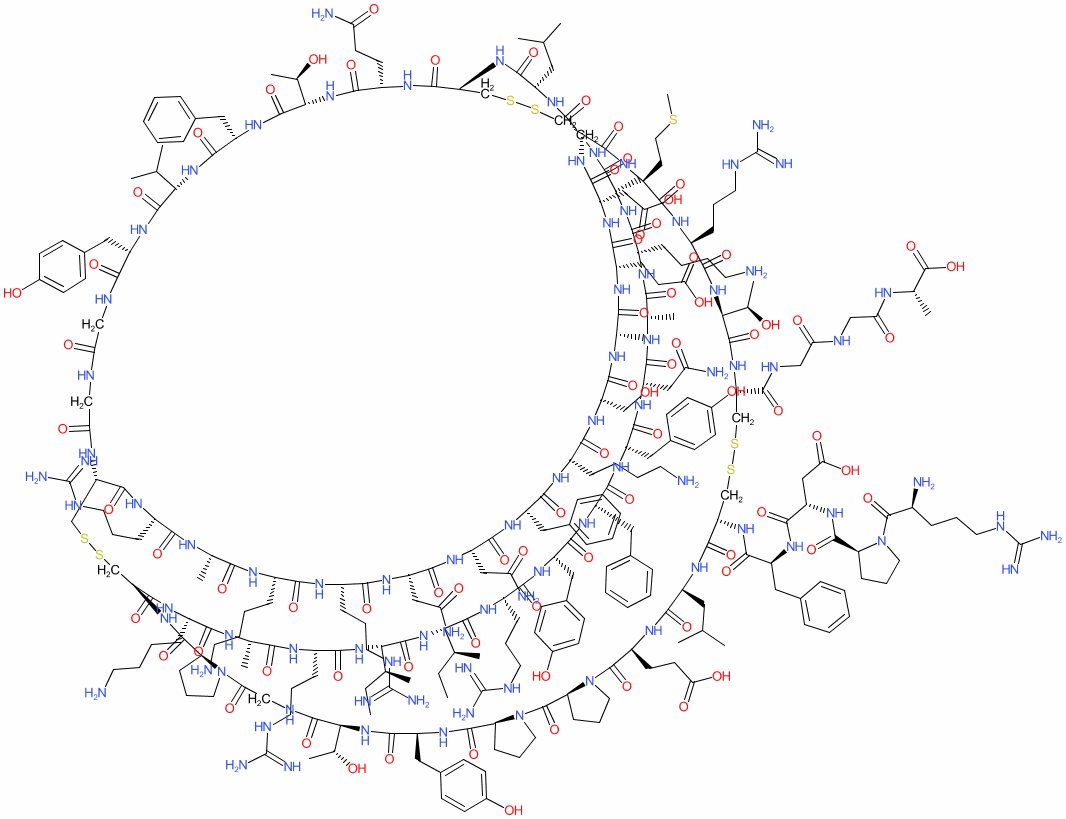#### GtoPdb Ligand ID: 6570

Synonyms: basic protease inhibitor (BPI) | BPTI | pancreatic trypsin inhibitor | Trasylol®
aprotinin is an approved drug (FDA (1993))
Comment: 58 amino-acid residue inhibitor of plasmin and other proteolytic enzymes.
Species: Bovine2D StructureSMILES / InChI / InChIKeyCanonical SMILES NCCCC[C@@H]1NC(=O)[C@@H]2CSSC[C@@H]3NC(=O)CNC(=O)CNC(=O)[C@H](Cc4ccc(cc4)O)NC(=O)[C@@H](NC(=O)[C@H](Cc4ccccc4)NC(=O)[C@@H](NC(=O)[C@H](CCC(=O)N)NC(=O)[C@H](CSSC[C@H](NC(=O)[C@H](CC(=O)O)NC(=O)[C@@H](NC(=O)[C@@H](NC(=O)[C@@H](NC(=O)[C@@H](NC(=O)[C@@H](NC(=O)[C@@H](NC(=O)[C@@H](NC(=O)[C@@H](NC(=O)[C@@H](NC(=O)[C@@H](NC(=O)[C@@H](NC3=O)CCCNC(=N)N)C)CCCCN)CCCNC(=N)N)CC(=O)N)CC(=O)N)Cc3ccccc3)CCCCN)CO)C)CCC(=O)O)C(=O)N[C@@H](CCSC)C(=O)N[C@H](C(=O)N[C@H](C(=O)N[C@@H](CSSC[C@@H](C(=O)N[C@H](C(=O)N[C@H](C(=O)N3[C@H](C(=O)N4[C@H](C(=O)N[C@H](C(=O)N[C@H](C(=O)NCC(=O)N5[C@H](C(=O)N2)CCC5)[C@H](O)C)Cc2ccc(cc2)O)CCC4)CCC3)CCC(=O)O)CC(C)C)NC(=O)[C@@H](NC(=O)[C@@H](NC(=O)[C@@H]2CCCN2C(=O)[C@H](CCCNC(=N)N)N)CC(=O)O)Cc2ccccc2)C(=O)NCC(=O)NCC(=O)N[C@H](C(=O)O)C)[C@H](O)C)CCCNC(=N)N)NC(=O)[C@H](CC(C)C)NC(=O)CNC(=O)[C@@H](NC(=O)[C@@H](NC(=O)[C@@H](NC(=O)[C@@H](NC(=O)[C@@H](NC(=O)[C@@H](NC(=O)[C@@H](NC(=O)[C@@H](NC(=O)[C@@H](NC(=O)[C@@H](NC(=O)[C@@H](NC(=O)[C@@H](NC1=O)C)CCCNC(=N)N)[C@H](CC)C)[C@H](CC)C)CCCNC(=N)N)Cc1ccc(cc1)O)Cc1ccccc1)Cc1ccc(cc1)O)CC(=O)N)C)CCCCN)C)[C@H](O)C)C(C)C Isomeric SMILES CC[C@H](C)[C@H]1C(=O)N[C@H](C(=O)N[C@H](C(=O)N[C@H](C(=O)N[C@H](C(=O)N[C@H](C(=O)N[C@H](C(=O)N[C@H](C(=O)N[C@H](C(=O)N[C@H](C(=O)NCC(=O)N[C@H](C(=O)N[C@H]2CSSC[C@H]3C(=O)N[C@H](C(=O)N[C@H](C(=O)N[C@H](C(=O)N[C@@H](CSSC[C@@H](C(=O)N[C@H](C(=O)N[C@H](C(=O)N4CCC[C@H]4C(=O)N4CCC[C@H]4C(=O)N[C@H](C(=O)N[C@H](C(=O)NCC(=O)N4CCC[C@H]4C(=O)N[C@@H](CSSC[C@@H](C(=O)N[C@H](C(=O)N[C@H](C(=O)N[C@H](C(=O)N[C@H](C(=O)N[C@H](C(=O)N[C@H](C(=O)N[C@H](C(=O)N[C@H](C(=O)N[C@H](C(=O)N[C@H](C(=O)N[C@H](C(=O)N[C@H](C(=O)N3)CC(=O)O)CCC(=O)O)C)CO)CCCCN)Cc3ccccc3)CC(=O)N)CC(=O)N)CCCNC(=N)N)CCCCN)C)CCCNC(=N)N)NC(=O)CNC(=O)CNC(=O)[C@@H](NC(=O)[C@@H](NC(=O)[C@@H](NC(=O)[C@@H](NC(=O)[C@@H](NC2=O)CCC(=O)N)[C@@H](C)O)Cc2ccccc2)C(C)C)Cc2ccc(cc2)O)C(=O)N[C@H](C(=O)N[C@H](C(=O)N[C@H](C(=O)N1)CCCNC(=N)N)C)CCCCN)[C@@H](C)O)Cc1ccc(cc1)O)CCC(=O)O)CC(C)C)NC(=O)[C@H](Cc1ccccc1)NC(=O)[C@H](CC(=O)O)NC(=O)[C@@H]1CCCN1C(=O)[C@H](CCCNC(=N)N)N)C(=O)NCC(=O)NCC(=O)N[C@@H](C)C(=O)O)[C@@H](C)O)CCCNC(=N)N)CCSC)CC(C)C)C)CCCCN)C)CC(=O)N)Cc1ccc(cc1)O)Cc1ccccc1)Cc1ccc(cc1)O)CCCNC(=N)N)[C@@H](C)CC InChI Key ZPNFWUPYTFPOJU-LPYSRVMUSA-N
 ClassificationCompound class Peptide or derivative Approved drug? Yes (FDA (1993))
 International Nonproprietary NamesINN number INN 2481 aprotinin
 Synonymsbasic protease inhibitor (BPI) | BPTI | pancreatic trypsin inhibitor | Trasylol®
Gene/PrecursorGene symbol Gene name Species Precursor protein name Synonyms
BPTI PTI pancreatic trypsin inhibitor [ Bos taurus (cattle) ] Bovine Pancreatic trypsin inhibitor -
 Database LinksCAS Registry No. 9087-70-1 (source: PubChem) DrugBank Ligand DB06692 GtoPdb PubChem SID 178103184 PubChem CID 16130295 Search Google for chemical match using the InChIKey ZPNFWUPYTFPOJU-LPYSRVMUSA-N Search Google for chemicals with the same backbone ZPNFWUPYTFPOJU Search PubMed clinical trials aprotinin Search PubMed titles aprotinin Search PubMed titles/abstracts aprotinin UniChem Compound Search for chemical match using the InChIKey ZPNFWUPYTFPOJU-LPYSRVMUSA-N UniChem Connectivity Search for chemical match using the InChIKey ZPNFWUPYTFPOJU-LPYSRVMUSA-N UniProtKB P00974 (Bt) Wikipedia Aprotinin